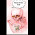## Wednesday, May 25, 2016

### Word Search for DEATH ON THE TREK

This book will be released June 13th, so I'd like to get you into the mood. Here's where it can be preordered if you're impatient:
(Let me know if you see it for preorder in other places, please.)

 H T U N A K E R T S S S Z M S D R U O G G P W A X L E Z W M O R O M W K L H N A T O D M G R L L A R F N A O L E E A V V I O K T A D T H O S R U A H C N Y T S W E N D A I E R R I A H C A M E T O E N G A E G A R E T D E N B M A P R R S N D E T E O A D I J A H Z W N F R Q F K E Z Y I T A G A S S N U V
 NEANDERTHAL GLACIER MAMMOTH DENISOVAN FIRE TREK STORYTELLER HAMAPA PRONOUNCEMENT DAKADAGA FIRETENDER SAGA ENGA TOG GOURDS

1.2.